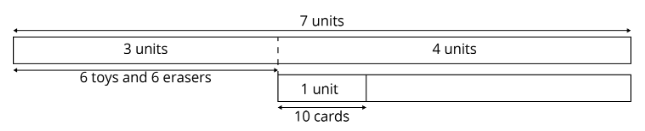Study P6 Mathematics Fractions Of Remainder - Geniebook# Fractions Of Remainder

In this article, we will focus on the word problems for fractions of a remainder.

## What is A Fraction of a Remainder?

Let’s look at this example on how we solve using models.

Freddy ate 14 of his birthday cake. He then cut the remaining cake into 6 equal pieces to give to his 6 friends. What fraction of the cake did each friend get?Fraction of cake remaining

\begin{align*}​ &= 1-​​\frac{1}{4}\\ &= \frac{3}{4} \end{align*}

The remaining \begin{align*}​ \frac{3}{4} \end{align*}of the cake was cut into $$6$$ equal pieces to give to his $$6$$ friends.

Fraction of cake each friend received

\begin{align*}​ &=\frac{3}{4} \div 6\\ &=\frac{3}{4} \div \frac{6}{1} \\ &=\frac{3}{4} \times \frac{1}{6} \\ &=\frac{1}{8} \end{align*}

\begin{align*}​ \frac{1}{8} \end{align*}

## Branching Method to Understand & Solve Problems

Branching method is also used to solve ’fraction of remainder’ questions. Let’s look at the examples below on how to solve using branching.

Question 1:

Joyce went shopping with $$560$$. She spent $$37$$ of the money on a pair of shoes and $$18$$ of the remaining money on a watch. She then bought a dress and had $$164$$ left.

How much did the dress cost?

Solution:Fraction representing the total amount of money spent on a dress and left

\begin{align*}​ &=\frac{4} {7} \times \frac{7}{8} \\ &=\frac{1}{2} \end{align*}

Total amount of money $$= 560$$

Total amount of money spent on a dress and left

\begin{align*}&= \frac{1}{2} \text{ of the total} \\ &= 560 \div 2 \\ &= 280​ \end{align*}

Cost of the dress

\begin{align*}​&= 280 - 164 \\ &= 116 \end{align*}

\begin{align*}​ 116 \end{align*}

Question 2:

Tom worked $$20$$ days in August. He gave $$0.15$$ of his salary to his mother. He spent $$45$$ of his remaining salary and saved the rest. He saved a total of $$340$$ in August. How much did he receive for a day’s work?

Solution:

\begin{align*}​ 0.15 &= \frac{15}{100}\\ &=\frac{3}{20} \end{align*}Fraction representing the amount of money saved

\begin{align*}&=\frac{1}{5} \times \frac{17}{20} \\ &=\frac{17}{100} \end{align*}

\begin{align*}​ \frac{17}{100} \end{align*} of the total $$= 340$$
\begin{align*}​ \frac{1}{100} \end{align*} of the total\begin{align*}​\\[2ex] &= 340 \div 17 \\[2ex] &= 20 \end{align*}
\begin{align*}​ \frac{100}{100} \end{align*} of the total\begin{align*}​\\[2ex] &= 100 \times 20 \\[2ex] & = 2000 \end{align*}

Amount of money he received for $$20$$ days of work $$= 2000$$a
Amount of money  he received  for a  day of work\begin{align*}​\\[2ex] &= 2000 \div 20 \\[2ex] &= 100 \end{align*}

$$100$$

Let’s look at the more challenging type of ‘fraction of a remainder’ questions.

Question 3:

Farmer Dan harvested some apples. He sold \begin{align*}​ \frac{3}{4} \end{align*} of the apples and additional $$105$$ apples to Adam. He sold $$55$$ less than \begin{align*}​ \frac{3}{4} \end{align*} of the remaining apples to Geraldine. Farmer Dan had $$890$$ apples left. How many apples did farmer Dan harvest?

Solution:\begin{align*}​ 1 \text{ part} &= 890 - 55 \\ &= 835 \\ \\ 4 \text{ part} &= 4 \times 835 \\ &= 3340 \end{align*}

From the model,

\begin{align*}​ 1 \text{ unit} &= 4 \text{ part} + 105 \\ &= 3340 + 105 \\ &= 3445 \end{align*}
Number of apples Farmer Dan harvested\begin{align*} \\[2ex] &= 4 \text{ units} \\ &= 4 \times 3445 \\ &= 13 \,780 \end{align*}

$$13 \,780$$ apples

Question 4:

Benjamin spent \begin{align*} \frac{3} {7} \end{align*} of his money on $$6$$ toys and $$6$$ erasers. He spent \begin{align*} \frac{1} {4} \end{align*}  of his remaining money on $$10$$ cards. Each eraser costs \begin{align*} \frac{1} {7} \end{align*} as much as a toy. Each card costs $$0.30$$ more than an eraser. How much money did Benjamin spend on each toy?

Solution:Since each eraser costs \begin{align*} \frac{1} {7} \end{align*} as much as a toy, we let the cost of $$1$$ eraser be represented by $$1$$ part and the cost of $$1$$ toy be represented by $$7$$ parts.

\begin{align*}​ \text{Cost of } 6 \text{ erasers} &= 6 \text{ parts} \\ \\ \text{Cost of } 6 \text{ toys} &= 6 \times 7 \text{ parts} \\ &= 42 \text{ parts} \\ \\ 3 \text{ units} &= 6 \text{ parts} + 42 \text{ parts} \\ &= 48 \text{ parts} \\ \\ 1 \text{ units} &= 48 \text{ parts} \div 3 \\ &= 16 \text{ parts} & \text{(Cost of 10 cards)} \end{align*}

Since each card costs $$0.30$$ more than an eraser,

\begin{align*}​ \text{Cost of } 10 \text{ cards} &= 10 \text{ parts} + 10 \times 0.30 \\ &= 10 \text{ parts} + 3 \\ \\ 16 \text{ parts} &= 10 \text{ parts} + 3 \end{align*}From the model,

\begin{align*}​ 6 \text{ parts} &= 3 \\ 1 \text{ parts} &= 3 \div 6 \\ &= 0.50 \\ \\ \text{Cost of }1 \text{ toy} &= 7 \text{ parts}\\ &= 7 \times 0.50\\ &= 3.50​ \end{align*}

$$3.50$$

## Conclusion

In this article, we worked on different word problems using the concept of fraction of the remainder.

We can solve such problems by different methods. We can draw models, use the branching method or the unitary method.

As always, practice, practice, and more practice will give you the much-needed confidence to tackle such problems in PSLE.

 Continue Learning Algebra Distance, Speed and Time Volume of Cubes and Cuboid Fundamentals Of Pie Chart Finding Unknown Angles Number Patterns: Grouping & Common Difference Fractions Of Remainder Fractions - Division Ratio Repeated Identity: Ratio StrategiesPrimaryPrimary 1Primary 2Primary 3Primary 4Primary 5Primary 6EnglishMaths
Algebra
Distance, Speed and Time
Volume of Cubes and Cuboid
Fundamentals Of Pie Chart
Finding Unknown Angles
Number Patterns: Grouping & Common Difference
Fractions Of Remainder
Fractions - Division
Ratio
Repeated Identity: Ratio StrategiesScienceSecondarySecondary 1Secondary 2Secondary 3Secondary 4